## Screenshots

•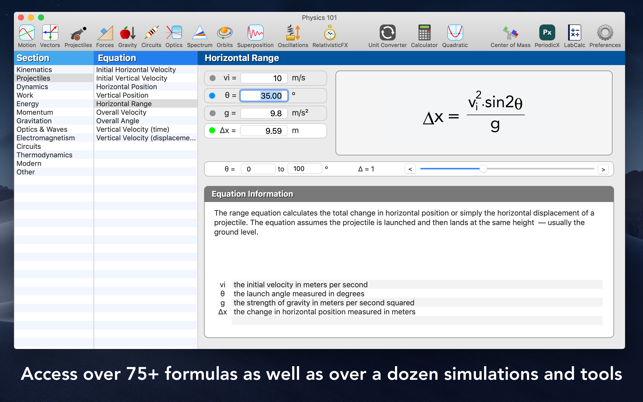•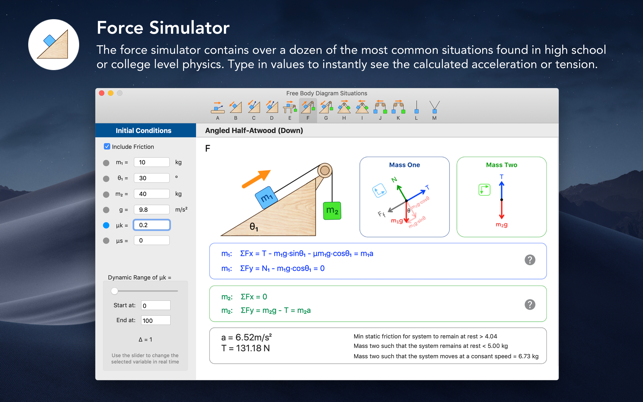•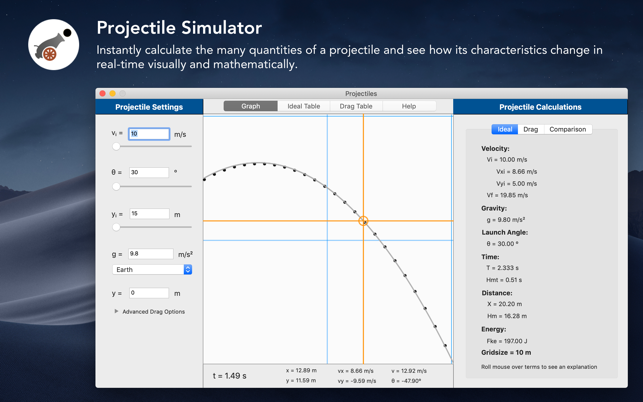•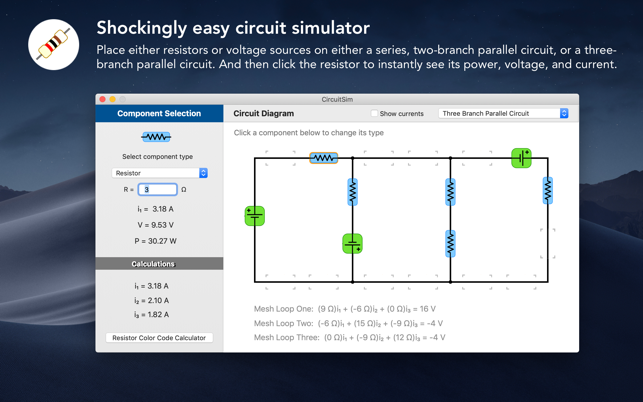•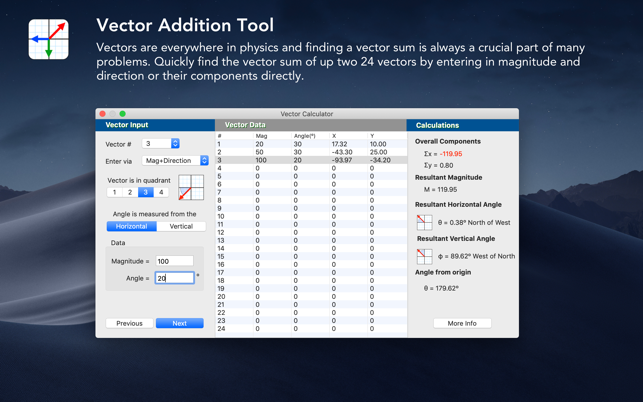•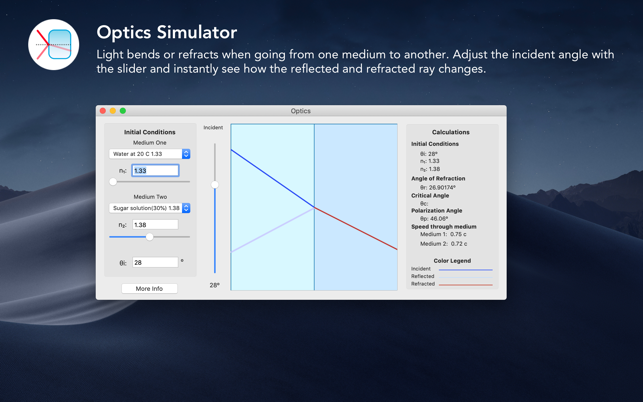•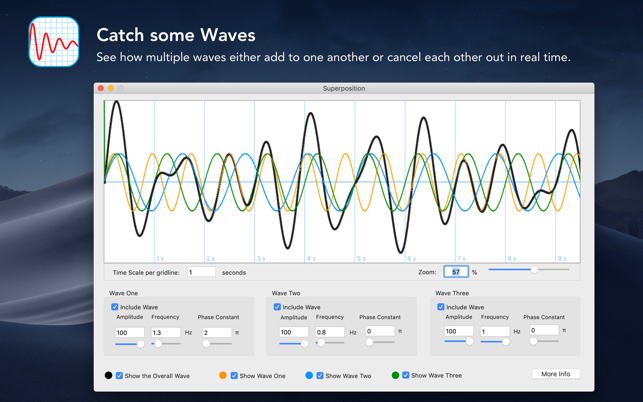•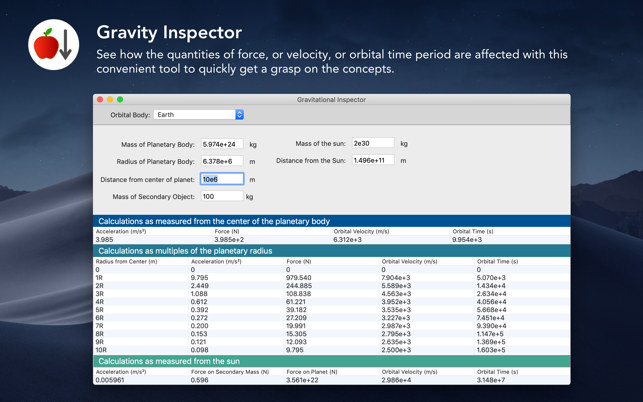•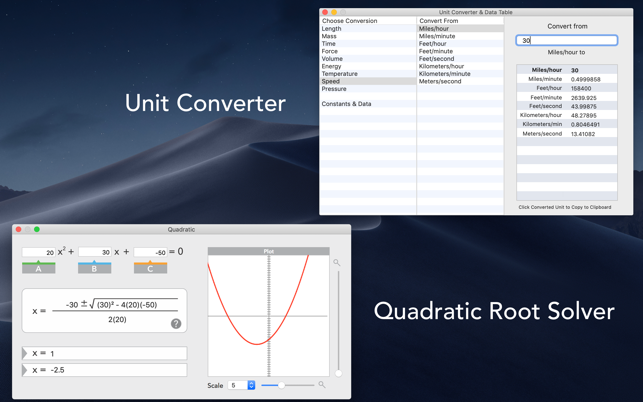## Beschreibung

Physics 101 contains over 75 equations and over a dozen simulations and tools covering the most important aspects of physics. Simulate a circuit, or launch a projectile, or work with the most common force diagram situations, and more. Whether you are a teacher wanting to augment your lessons or a student wanting better grasp of the material, Physics 101 is your go-to solution.

Physics is more than just a numerical answer — it is about understanding the rich nature behind the equations. Each formula explains its purpose and lists out an explanation for each of the variables used. Since Physics 101 lets you calculate values on the fly you can start to uncover the subtle and nuanced nature of the equations as you manipulate values in the formulas and simulations.

Major features include:

• 75+ Physics Equations organized in over a dozen section
• Circuit Analysis Simulator
• Force Simulator
• Lab Report
• Motion Analysis Feature
• Optics Simulator
• Orbits simulator
• Oscillations
• Periodic Table
• Printable Formula Guides
• Projectile Simulator
• QuickCal
• RelativisticFX
• Superposition
• Unit Converter

## Neuheiten

Version 9.0.2

Physics 101 has been totally refinished end-to-end with a new real-time equation interface and every major tool and simulation has been refined, enhanced, and modernized. It is the biggest update ever.

4.3 von 5
3 Bewertungen

3 Bewertungen

## Informationen

Anbieter
Jake Mavity
Größe
22.9 MB
Kategorie
Bildung
Kompatibilität

OS X 10.10.0 oder neuer, 64-Bit-Prozessor

Sprachen

Englisch

Alter
4+
•